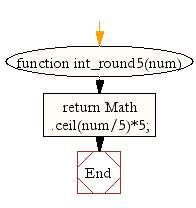# JavaScript: Round up an integer value to the next multiple of 5

## JavaScript Math: Exercise-28 with Solution

Write a JavaScript function to round up an integer value to the next multiple of 5.

Test Data:
console.log(int_round5(32));
35
console.log(int_round5(137));
140

Sample Solution:-

HTML Code:

``````<!DOCTYPE html>
<html>
<meta charset="utf-8">
<title>JavaScript function to round up an integer value to the next multiple of 5</title>
<body>

</body>
</html>
```
```

JavaScript Code:

``````function int_round5(num)
{
return Math.ceil(num/5)*5;
}

console.log(int_round5(32));
console.log(int_round5(137));
console.log(int_round5(142));
```
```

Sample Output:

```35
140
145
```

Pictorial Presentation:Flowchart:Live Demo:

See the Pen javascript-math-exercise-28 by w3resource (@w3resource) on CodePen.

Improve this sample solution and post your code through Disqus

What is the difficulty level of this exercise?

Test your Programming skills with w3resource's quiz.

﻿